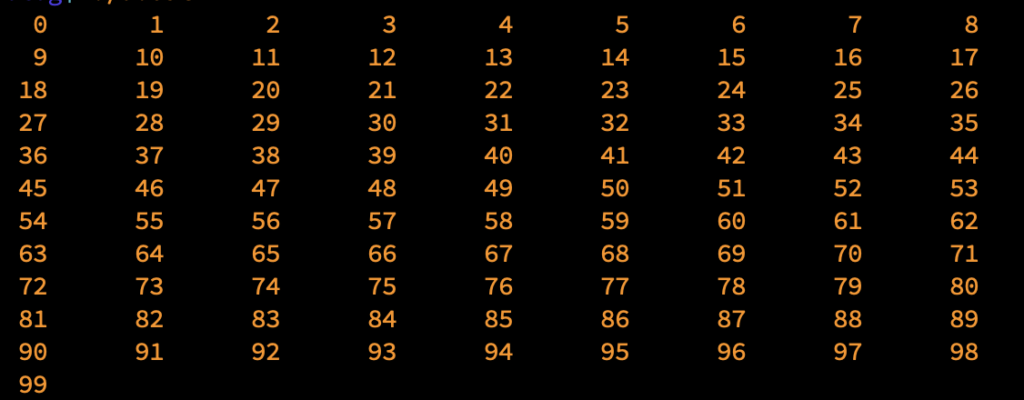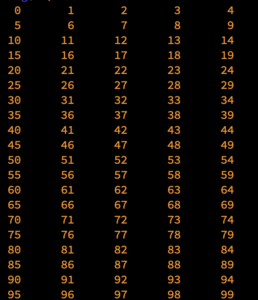# Generating a Table from Stream Output

This month’s Exercise searched for and output the first 100 cyclops numbers. But instead of spewing them out in a long column, my solution set them in a table. The values marched across the screen in neat rows. This trick is rather easy to accomplish, but in my solution I wanted some flexibility with the column number.

I took code from the relevant part of my Exercise solution and rebuilt it here to output sequential values:

### 2022_09_10-Lesson.c

```#include <stdio.h>

int main()
{
const int columns = 9;
const int values = 100;
int x,y,count;

x = 0;
count = values;
while( count )
{
for( y=0; y<columns; y++ )
{
printf("%3d",(x*columns)+y );
count--;                /* decrement the count */
if( !count )            /* if count is zero, stop */
break;
if( y<columns-1)
putchar('\t');
}
x++;
putchar('\n');
}

return(0);
}```

The number of `columns` is set as a constant, as is the `values` count. I wanted the table output to handle a variable number of columns, but also to deal with a situation when the last row doesn’t have a value for each column.

The nested for loop handles the values across a row. The key to outputting the values sequentially is found in the printf() statement: `(x*columns)+y)` This expression bases each row’s values on variable `x`, which is the row count, times the total number of columns. Adding the `y` value completes the task, as each value is output sequentially, left-to-right, top-down, as shown in Figure 1.Figure 1. The output showing 100 values in nine columns.

The expression `x*columns` increments the starting (first column) value by the value of columns: 0, 9, 18, and so on. The rest of the row then falls into place based on the value of variable `y`.

This code is constructed so that the column number can be adjusted without requiring the loops to be rebuilt. If I set the value of constant `columns` to five, the output changes as shown in Figure 2.Figure 2. Table output when the `columns` constant is set to five.

When the rows and columns are even, as shown in Figure 2, the last row is full. But in Figure 1, you see only one item on the final row. The code that handles this is an if decision after the printf() statement outputs the value:

```if( !count )     break;```

Variable `count` is decremented after output. If its value is zero, values are exhausted and the row can end: The for loop stops and a newline is output. Because `count` is zero, the outer while loop stops as well.

Table output raises the difficulty level over just spewing out each number on a line by itself. This method, rows across, is easier than coding a table where the values are set in columns marching left-to-right. I cover this type of table output in next week’s Lesson.